# Class 9 RD Sharma Solutions – Chapter 16 Circles- Exercise 16.4

• Last Updated : 04 May, 2021

### Question 1. In Fig., O is the centre of the circle. If ∠APB∠APB= 50°, find ∠AOB and ∠OAB.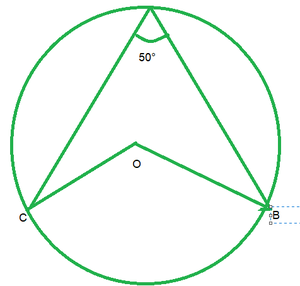Solution:

Hey! Looking for some great resources suitable for young ones? You've come to the right place. Check out our self-paced courses designed for students of grades I-XII

Start with topics like Python, HTML, ML, and learn to make some games and apps all with the help of our expertly designed content! So students worry no more, because GeeksforGeeks School is now here!

∠APB=50°

By degree measure theorem

∠AOB=2APB

∠APB=2*50°=100°

Then ∠OAB=∠OBA            [Angles opposite to equal sides]

Let ∠OAB=x

In △OAb, by angle sum property

∠OAB+∠OBA+∠AOB=180°

x+x+100°=180°

2x=180°-100°

2x=80°

x=40°

∠OAB=∠OBA=40°

### Question 2. In Fig., it is given that O is the centre of the circle and ∠AOC = 150°. Find ∠ABC.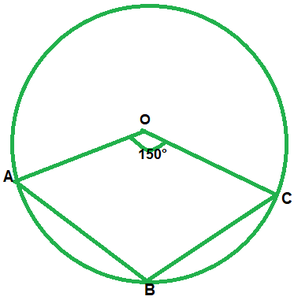Solution:

∠AOC = 150°

∠AOC +reflex ∠AOC = 360°             [complex angle]

150°+reflex ∠AOC = 360°

reflex ∠AOC=210°

2∠ABC=210°          [By degree measure theorem]

∠ABC=210°/2=105°

### Question 3. In Fig., O is the centre of the circle. Find ∠BAC.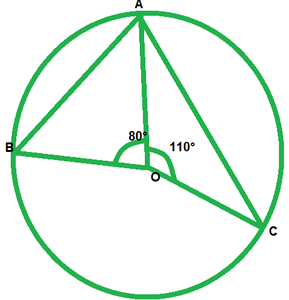Solution:

We have ∠AOB=80°

And ∠AOC=110°

Therefore, ∠AOB+∠AOC+∠BOC=360°          [complete angle]

80+100+∠BOC=360°

∠BOC=360°-80°-110°

∠BOC=70°

By degree measure theorem

∠BOC=2∠BAC

170=2∠BAC

∠BAC=170°/2=85°

### Question 4. If O is the centre of the circle, find the value of x in each of the following figures.

Solution:

i)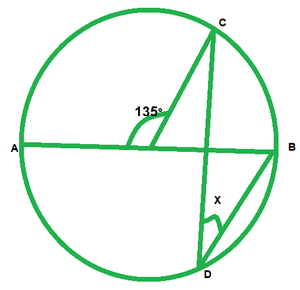∠AOC=135°

∠AOC+BOC=185°             [Linear pair of angles]

135°+∠BOC=180°

∠BOC=180°-135°=45°

By degree measure theorem

∠BOC=2∠COB

45=2x

x=45°/2=22\frac{1}{2}

ii)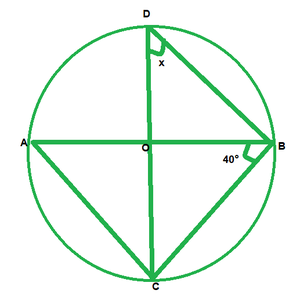We have

∠ABC=40°

∠ACB=90°               [Angle in semicircle]

In △ABC, by angle sum property

∠CAB+∠ACB+∠ABC=180°

∠CAB+90°+40°=180°

∠CAB=180°-90°-40°

∠CAB=50°

Now,

∠CDB=∠CAB         [Angle is same in segment]

x=50°

iii)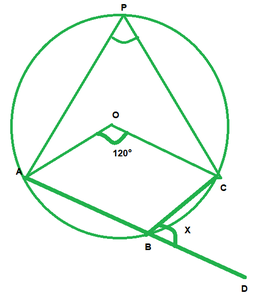We have,

∠AOC=120°

By degree measure theorem

∠AOC=2∠APC

120°=2∠APC

∠APC=120°/2=60

∠APC+∠ABC=180°      [Opposite angles of cyclic quadrilaterals]

60°+∠ABC=180°

∠ABC=180°-60°

∠ABC=120°

∠ABC+∠DBC=180°      [Linear pair of angles]

120°+x=180°

x=180°-120°=60°

iv)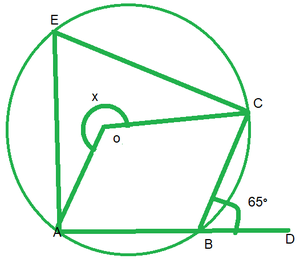We have

∠CBD=65°

∠ABC+∠CBD=180°      [Linear pair of angles]

∠ABC=65°=180°

∠ABC=180°-65°=115°

reflex ∠AOC=2∠ABC          [By degree measure theorem]

x=2*115°

x=230°

v)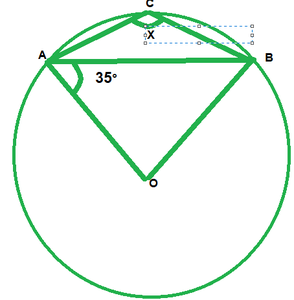We have,

∠OAB=35°

Then, ∠OBA=∠OAB=35°      [Angles opposite to equal radii]

In △AOB, by angle sum property

∠AOB+∠OAB+∠OBA=180°

∠AOB+35°+35°=180°

∠AOB=180°-35°=110°

∠AOB+reflex ∠AOB=360°             [complex angle]

110+reflex∠AOB=360°

reflex∠AOB=360°-110°=250°

By degree measure theorem reflex∠AOB=2∠ACB

250°=2x

x=250°/2=125°

vi)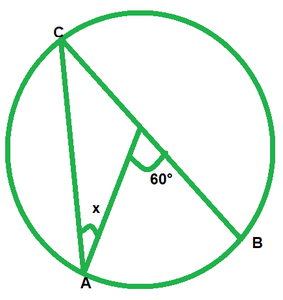We have,

∠AOB=60

By degree measure theorem reflex

∠AOB=2∠ACB

60=2∠ACB

∠ACB=60°/2=30°         [Angle opposite to equal radii]

x=30°

vii)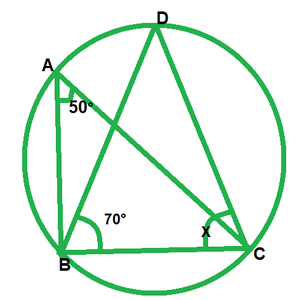We have,

∠BAC=50°  and  ∠DBC=70°

∠BDC=∠BAC=50°           [Angle in same segment]

In △BDC, by angle sum property

∠BDC+∠BCD+∠DBC=180°

50°+x+70°=180°

x=180°-50°-70°=60°

viii)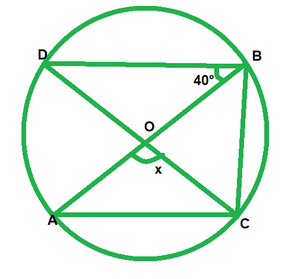We have,

∠DBO=40° and ∠DBC=90°        ——-[Angle in a semi circle]

∠DBO+∠OBC=90°

40°+∠OBC=90°

∠OBC=90°-40°=50°

By degree measure theorem

∠AOC=∠OBC

x=2*50°=100°

ix)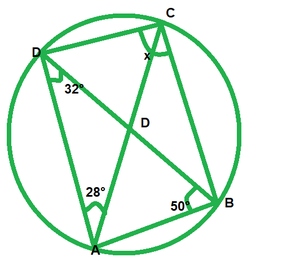In ∆DAB, by angle sum property

32°+∠DAB+50°=180°

∠DAB=180°-32°-50°

∠DAB=98°

Now,

∠OAB+∠DCB=180°          [opposite angle of cyclic quadrilateral]

98°+x=180°

x=180°-98°=82°

x)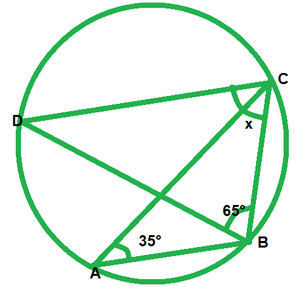We have,

∠BAC=35°

∠BDC=∠BAC=35°            [Angle in same segment]

In ∆BCD, by angle sum property

∠BDC+∠BCD+∠DBC=180°

35°+x+65°=180°

x=180°-35°-65°=80°

xi)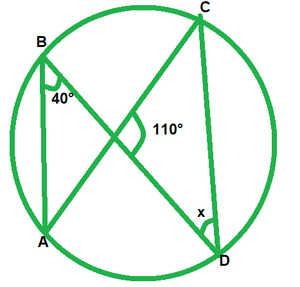We have,

∠ABD=40°

∠ACD=∠ABD=40°         [Angle in same segment]

In ∆PCD, by angle sum property

∠PCD+∠CPO+∠PDC=180°

40°+110°+x=180°

x=180°-150°

x=30°

xii)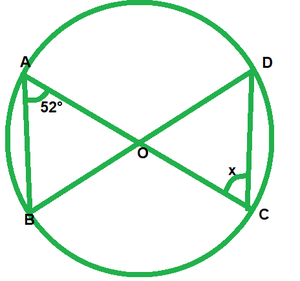Given that,

∠BAC=52°

Then ∠BDC=∠BAC=52°        [Angle in same segment]

Since OD=OC

Then ∠ODC=∠OCD            [ Opposite angle to equal radii]

x=52°

### Question 5. O is the circumcenter of the triangle ABC and OD is perpendicular on BC. Prove that ∠BOD = ∠A.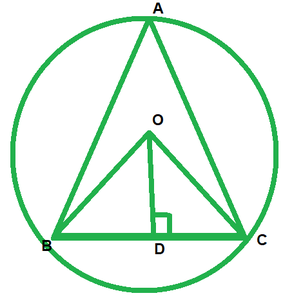Solution:

We have to prove that  ∠BOD=∠A

since, circum center  is the intersection of perpendicular bisector of each side of the the triangle. Now according to figure A,B,C are the vertices of ∆ABC

In ∆BOC, OD is perpendicular  bisector  of BC.

so, BD=CD

OB=OC        ——–(Radius of the same circle)

And,

OD=OD            —–[common]

Therefore,

∆BDO≅∆CDO              (SSS concurrency criterion )

∠BOD=∠COD              (by cpct)

We know that angle formed any chord  of the circle at the center is twice of the  angle formed at  the circumference  by same chord

Therefore,

∠BAC=\frac{1}{2}  ∠BOC

∠BAC=\frac{1}{2}*2∠BOD

∠BAC=∠BOD

Therefore,

∠BOD=∠A

### Question 6. In Fig., O is the centre of the circle, BO is the bisector of ∠ABC. Show that AB = AC.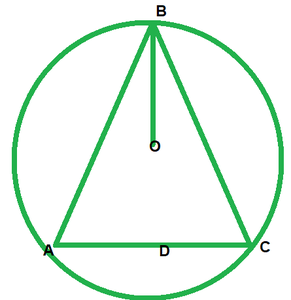Solution:

Given, BO is the bisector of ∠ABC

To prove: AB=BC

Proof:  Since, BO is the bisector  of ∠ABC.

Then, ∠ABO=∠CBO        —-(i)

Then, ∠ABO=∠DAB           ——–(ii)    [opposite angles to equal sides]

Then, ∠OAB=∠OCB       ——–(iii)  [opposite angles to equal sides]

compare equations  (i), (ii) and (iii)

∠OAB=∠OCB ——-(iv)

In ∆OAB  and   ∆OCB

∠OAB=∠OCB       [From(iv)]

∠OBA=∠OBC          [Given]

OB=OB           [common]

Then

∆OAB≅∆OCB        [By AAS condition]

Therefore, AB=BC      [CPCT]

### Question 7. In Fig., O is the centre of the circle, prove that ∠x = ∠y + ∠z.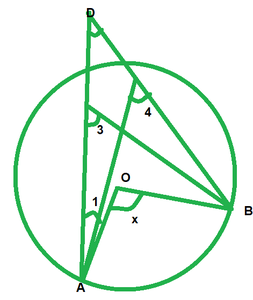Solution:

We have,

∠3=∠4            [Angles in same segment]

∠x=2∠3          [By degree measure theorem]

∠x=∠3+∠3⇒∠x=∠3+∠4 ——–(i)        [∠3=angle 4]

But ∠y=∠3+∠1          [By exterior angle property]

⇒∠3=∠y-∠1    —-(ii)

from (i) and (ii)

∠x=∠y-∠1+∠4

∠x=∠y+∠4-∠1

∠x=∠y+∠z+∠1-∠1           [By exterior angle property]

∠x=∠y+∠z

### Question 8. In Fig., O and O’ are centres of two circles intersecting at B and C. ACD is a straight line, find x.Solution:

By degree measure theorem

∠AOB=2∠ACB

130°=2∠ACB⇒∠ACB=130°/2=65

∠ACB+∠BCD=180°        [Linear pair of angles]

65°+∠BCD=180°

∠BCD=180-65=115

By degree measure theorem

reflex∠BOD=2∠BCD

reflex∠BOD=2*115°=230°

Now, reflex∠BOD+∠BOD=360°       [complex angle]

230°+x=360°

x=360°-230°

x=130°

### Question 9. In Fig., O is the centre of a circle and PQ is a diameter. If ∠ROS = 40°, find ∠RTS.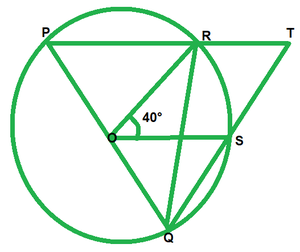Solution:

Since PQ is diameter

Then,

∠PRQ=90°          [Angle in semi circle]

∠PRQ+∠TRQ=180°           [Linear pair of angle]

90+∠TRQ=180

∠TRQ=180°-90°=90°

By degree measure  theorem

∠ROS=2∠RQS

40=2∠RQS

∠RQS=40°/2=20°

In ∆RQT, by angle  sum property

∠RQT+∠QRT+∠RTS=180°

20°+90°+∠RTS=180°

### Question 10. In Fig., if ∠ACB = 40°, ∠DPB = 120°, find ∠CBD.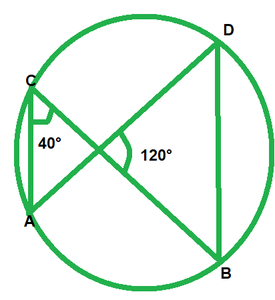Solution:

We have,

∠ACB=40°;  ∠DPB=120°

∠APB=∠DCB=40°            [Angle in same segment]

In ∆POB, by angle sum property

∠PDB+∠PBD+∠BPD=180°

40+∠PBD+120°=180°

∠PBD=180°-40°-120°

∠PBD=20°

∠CBD=20°

### Question 11. A chord of a circle is equal to the radius of the circle. Find the angle subtended by the chord at a point on the minor arc and also at a point on the major arc.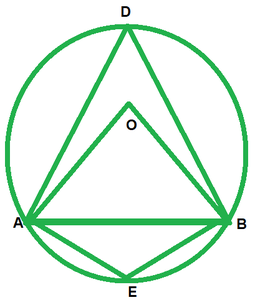Solution:

Construction: O is center and r is radius and given that chord is equal to radius of circle.

Now in ∆AOB we have

AO=OB=BA         (It is given that chord is equal to radius of circle)

so, ∆AOB is an equilateral triangle

∠AOB=60°

So, ∠AOB=2∠ADB     (The angle subtended by an arc of a circle at the center  is double the angle subtended by it at any point  on the remaining  prat of the circle)

So,Therefore,